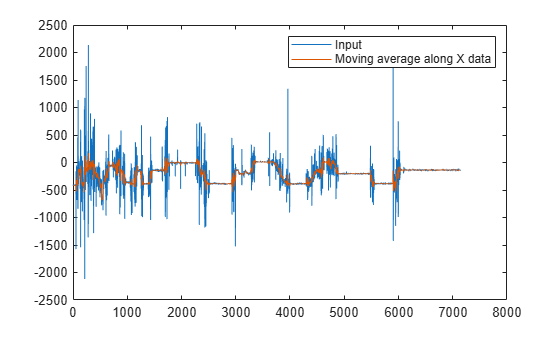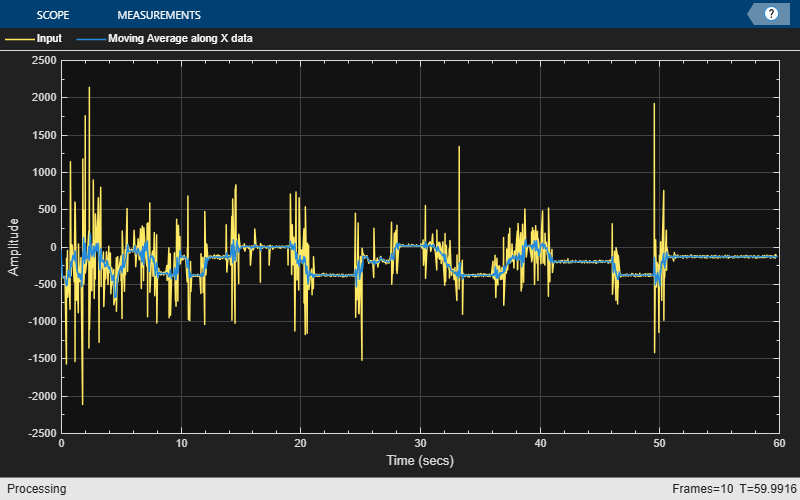## Measure Statistics of Streaming Signals

The moving statistics System objects measure statistics of streaming signals in MATLAB®. You can also use functions such as `movmean`, `movmedian`, `movstd`, and `movvar` to measure the moving statistics. These functions are more suitable for one-time computations on data that is available in a batch. Unlike System objects, the functions are not designed to handle large streams of data.

### Compute Moving Average Using Only MATLAB Functions

This example shows how to compute the moving average of a signal using the `movmean` function.

The `movmean` function computes the 10-point moving average of the noisy data coming from an accelerometer. The three columns in this data represent the linear acceleration of the accelerometer in the X-axis, Y-axis, and Z-axis, respectively. All the data is available in a MAT file. Plot the moving average of the X-axis data.

```winLen = 10; accel = load('LSM9DS1accelData73.mat'); movAvg = movmean(accel.data,winLen,'Endpoints','fill'); plot([accel.data(:,1),movAvg(:,1)]); legend('Input','Moving average along X data');```The data is not very large (7140 samples in each column) and is entirely available for processing. The `movmean` function is designed to handle such one-time computations. However, if the data is very large, such as in the order of GB, or if the data is a live stream that needs to be processed in real time, then use System objects. The System objects divide the data into segments called frames and process each frame in an iteration loop seamlessly. This approach is memory efficient, because only one frame of data is processed at any given time. Also, the System objects are optimized to handle states internally.

### Compute Moving Average Using System Objects

Create a `dsp.MovingAverage` System object? to compute the 10-point moving average of the streaming signal. Use a `dsp.MatFileReader` System object to read data from the accelerometer MAT file. View the moving average output in the time scope.

The System objects automatically index the data into frames. Choose a frame size of 714 samples. There are 7140 samples or 10 frames of data in each column of the MAT file. Each iteration loop computes the moving average of 1 frame of data.

```frameSize = 714; reader = dsp.MatFileReader('SamplesPerFrame',frameSize,... 'Filename','LSM9DS1accelData73.mat','VariableName','data'); movAvg = dsp.MovingAverage(10); scope = dsp.TimeScope('NumInputPorts',2,'SampleRate',119,'YLimits',[-2500 2500],... 'ChannelNames',{'Input','Moving Average along X data'},'TimeSpan',60,'ShowLegend',true); while ~isDone(reader) accel = reader(); avgData = movAvg(accel); scope(accel(:,1),avgData(:,1)); end ```The processing loop is very simple. The System Objects handle data indexing and states automatically.

Watch now